# Gauss' Law for Electricity

The electric flux out of any closed surface is proportional to the total charge enclosed within the surface.

The integral form of Gauss' Law finds application in calculating electric fields around charged objects.

In applying Gauss' law to the electric field of a point charge, one can show that it is consistent with Coulomb's law.

While the area integral of the electric field gives a measure of the net charge enclosed, the divergence of the electric field gives a measure of the density of sources. It also has implications for the conservation of charge.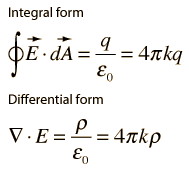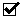Gauss' law, electricity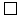Gauss' law, magnetismFaraday's lawAmpere's law
 Maxwell's Equations
Index

Maxwell's equations concepts

 HyperPhysics***** Electricity and Magnetism R Nave
Go Back

# Gauss' Law for Magnetism

The net magnetic flux out of any closed surface is zero. This amounts to a statement about the sources of magnetic field. For a magnetic dipole, any closed surface the magnetic flux directed inward toward the south pole will equal the flux outward from the north pole. The net flux will always be zero for dipole sources. If there were a magnetic monopole source, this would give a non-zero area integral. The divergence of a vector field is proportional to the point source density, so the form of Gauss' law for magnetic fields is then a statement that there are no magnetic monopoles.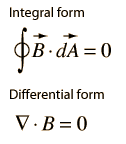Gauss' law, electricityGauss' law, magnetismFaraday's lawAmpere's law
 Maxwell's Equations
Index

Maxwell's equations concepts

 HyperPhysics***** Electricity and Magnetism R Nave
Go Back

The line integral of the electric field around a closed loop is equal to the negative of the rate of change of the magnetic flux through the area enclosed by the loop.

This line integral is equal to the generated voltage or emf in the loop, so Faraday's law is the basis for electric generators. It also forms the basis for inductors and transformers.

 Application to voltage generation in a coil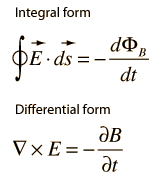Gauss' law, electricityGauss' law, magnetismFaraday's lawAmpere's law
 Maxwell's Equations
Index

Maxwell's equations concepts

 HyperPhysics***** Electricity and Magnetism R Nave
Go Back

# Ampere's Law

In the case of static electric field, the line integral of the magnetic field around a closed loop is proportional to the electric current flowing through the loop. This is useful for the calculation of magnetic field for simple geometries.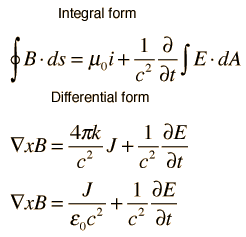Gauss' law, electricityGauss' law, magnetismFaraday's lawAmpere's law
 Apply to charge conservation
 Maxwell's Equations
Index

Maxwell's equations concepts

 HyperPhysics***** Electricity and Magnetism R Nave
Go Back